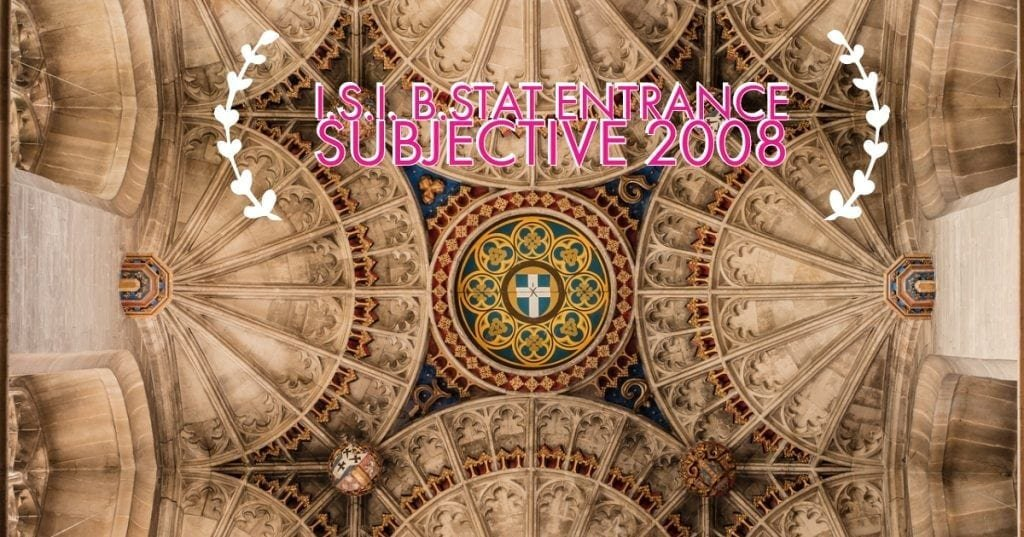How Cheenta works to ensure student success?
Explore the Back-Story

# ISI B.Stat 2008 Subjective Paper| Problems & SolutionsHere, you will find all the questions of ISI Entrance Paper 2008 from Indian Statistical Institute's B.Stat Entrance. You will also get the solutions soon of all the previous year problems.

Problem 1:

Of all triangles with given perimeter, find the triangle with the maximum area. Justify your answer

Problem 2:

A $40$ feet high screen is put on a vertical wall $10$ feet above your eye-level. How far should you stand to maximize the angle subtended by the screen (from top to bottom) at your eye?

Problem 3:

Study the derivatives of the function
$\mathbf{y=\sqrt{x^3-4x}}$
and sketch its graph on the real line.

Problem 4:

Suppose $P$ and $Q$ are the centres of two disjoint circles $\mathbf{C_1}$ and $\mathbf{C_2}$ respectively, such that $P$ lies outside $\mathbf{C_2}$ and $Q$ lies outside $\mathbf{C_1}$. Two tangents are drawn from the point $P$ to the circle $\mathbf{C_2}$, which intersect the circle $\mathbf{C_1}$ at point $A$ and $B$. Similarly, two tangents are drawn from the point $Q$ to the circle $\mathbf{C_1}$, which intersect the circle $\mathbf{C_2}$ at points $M$ and $N$. Show that $AB=MN$

Problem 5:

Suppose $ABC$ is a triangle with inradius $r$. The incircle touches the sides $BC$, $CA$, and $AB$ at $D,E$ and $F$ respectively. If $BD=x$, $CE=y$ and $AF=z$, then show that $\mathbf{r^2=\frac{xyz}{x+y+z}}$

Problem 6:

Evaluate: $\lim_{n \to\infty} \frac{1}{2n} \ln {{2n} \choose{n}}$

Problem 7:

Consider the equation $\mathbf{x^5+x=10}$. Show that
(a) the equation has only one real root;
(b) this root lies between $1$ and $2$;
(c) this root must be irrational.

Problem 8:

In how many ways can you divide the set of eight numbers $\mathbf{{2,3,\cdots,9}}$ into $4$ pairs such that no pair of numbers has $\mathbf{\text{gcd} }$ equal to $2$?

Problem 9:

Suppose $S$ is the set of all positive integers. For $\mathbf{a,b \in S}$, define
$\mathbf{a * b=\frac{\text{lcm}[a,b]}{\text{gcd}(a,b)}}$
For example $8 * 12=6$.
Show that exactly two of the following three properties are satisfied:
(i) If $\mathbf{a,b \in S}$, then $\mathbf{a * b \in S}$.
(ii) $\mathbf{(a*b)*c=a*(b*c)}$ for all $\mathbf{a,b,c \in S}$.
(iii) There exists an element $\mathbf{i \in S}$ such that $\mathbf{a *i =a}$ for all $\mathbf{a \in S}$

Problem 10:

Two subsets $A$ and $B$ of the ($x,y$)-plane are said to be equivalent if there exists a function $f: A$ to $B$ which is both one-to-one and onto.
(i) Show that any two line segments in the plane are equivalent.
(ii) Show that any two circles in the plane are equivalent.

Here, you will find all the questions of ISI Entrance Paper 2008 from Indian Statistical Institute's B.Stat Entrance. You will also get the solutions soon of all the previous year problems.

Problem 1:

Of all triangles with given perimeter, find the triangle with the maximum area. Justify your answer

Problem 2:

A $40$ feet high screen is put on a vertical wall $10$ feet above your eye-level. How far should you stand to maximize the angle subtended by the screen (from top to bottom) at your eye?

Problem 3:

Study the derivatives of the function
$\mathbf{y=\sqrt{x^3-4x}}$
and sketch its graph on the real line.

Problem 4:

Suppose $P$ and $Q$ are the centres of two disjoint circles $\mathbf{C_1}$ and $\mathbf{C_2}$ respectively, such that $P$ lies outside $\mathbf{C_2}$ and $Q$ lies outside $\mathbf{C_1}$. Two tangents are drawn from the point $P$ to the circle $\mathbf{C_2}$, which intersect the circle $\mathbf{C_1}$ at point $A$ and $B$. Similarly, two tangents are drawn from the point $Q$ to the circle $\mathbf{C_1}$, which intersect the circle $\mathbf{C_2}$ at points $M$ and $N$. Show that $AB=MN$

Problem 5:

Suppose $ABC$ is a triangle with inradius $r$. The incircle touches the sides $BC$, $CA$, and $AB$ at $D,E$ and $F$ respectively. If $BD=x$, $CE=y$ and $AF=z$, then show that $\mathbf{r^2=\frac{xyz}{x+y+z}}$

Problem 6:

Evaluate: $\lim_{n \to\infty} \frac{1}{2n} \ln {{2n} \choose{n}}$

Problem 7:

Consider the equation $\mathbf{x^5+x=10}$. Show that
(a) the equation has only one real root;
(b) this root lies between $1$ and $2$;
(c) this root must be irrational.

Problem 8:

In how many ways can you divide the set of eight numbers $\mathbf{{2,3,\cdots,9}}$ into $4$ pairs such that no pair of numbers has $\mathbf{\text{gcd} }$ equal to $2$?

Problem 9:

Suppose $S$ is the set of all positive integers. For $\mathbf{a,b \in S}$, define
$\mathbf{a * b=\frac{\text{lcm}[a,b]}{\text{gcd}(a,b)}}$
For example $8 * 12=6$.
Show that exactly two of the following three properties are satisfied:
(i) If $\mathbf{a,b \in S}$, then $\mathbf{a * b \in S}$.
(ii) $\mathbf{(a*b)*c=a*(b*c)}$ for all $\mathbf{a,b,c \in S}$.
(iii) There exists an element $\mathbf{i \in S}$ such that $\mathbf{a *i =a}$ for all $\mathbf{a \in S}$

Problem 10:

Two subsets $A$ and $B$ of the ($x,y$)-plane are said to be equivalent if there exists a function $f: A$ to $B$ which is both one-to-one and onto.
(i) Show that any two line segments in the plane are equivalent.
(ii) Show that any two circles in the plane are equivalent.

This site uses Akismet to reduce spam. Learn how your comment data is processed.

### 3 comments on “ISI B.Stat 2008 Subjective Paper| Problems & Solutions”

1.Spandan says:

How to solve problem number 5 and 6?

1.dev kit says:

Solution to 6: Let y be the given limit. Then e^y = (2nCn)^(1/2n). Now 2nCn = (2n)!/(n!)^2 . We divide both Nr and Dr by (2n)^2n. Hence e^y = [ {(2n)!/(2n)^2n}^(1/2n)]/[{n!/n^n}^2]^(1/2n)*2. Now limit n tends to infinity (n!/n^n)^(1/2n) is e^-1. Hence on simplification limit n tends to infinity e^y =2 and finally result is log 2. Do it yourself to understand the solution.

1.arvind says:

you can expand the factorial and use definite integral as a sum of infinite terms. the things multiplied in the log can be split and then take as a summation which can be used as an integral by putting r/n as x and 1/n as dx

### Knowledge Partner